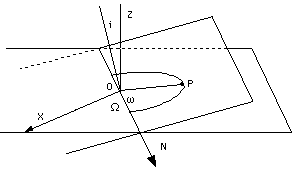# Disclaimer: The following material is being kept online for archival purposes.

Site Map

## Added note, especially for those who have studied section (12a) "How Orbital Motion is Calculated"

 The simple pair of formulas derived in problem (8) x' = x cosα – y sinα y' = x sinα + y cosα is very powerful and useful. For instance, it can reproduce rotations in 3-dimensional space by first rotating (x,y) by some angle α around the z axis,, giving the above result and producing (x',y'), while z remains the same, so that in the new system z' = z. Then we rotate (x',y',z') by an angle β around the x' axis, using the same formulas but with (y',z', β) in place of (x,y, α). Let the new variables be (x",y",z"). Then x' remains the same, i.e. x"=x', but (y',z') are replaced by y" = y' cosβ – z' sinβ z" = y' sinβ + z' cosβ Suppose a satellite's position is given by its orbital elements. Knowing its semi-major axis a, eccentricity e and mean anomaly M, one can derive its true anomaly f (using appropriate formulas, not given in #12a) and from it the radial distance r r = a(1 – e2)/(1 + e cosf) In cartesian coordinates (x',y',z'), with z' perpendicular to the orbital plane and x' along the major axis, the satellite's position is x' = r cosf         y' = r sinf         z' = 0But what are its actual celestial coordinates (x,y,z)? From the illustration shown here (taken from section #12a), (x,y,z) can be reached by conducting two simple rotations by angles (ω, i, Ω). To connect the two systems, the simple transformation derived above is applied, consecutively--first a rotation around line N by an angle i giving an intermediate system (x",y",z"), after which z' = z" = z. And then another rotation by an angle ω+Ω around the shared z axis, bringing the x' axis, which originally passed the apogee P, into the celestial x axis. Special notations exist which simplify the job. Problems involving the orientation of satellites in 3-dimensional space (the "attitude" of those satellites) are handled in a similar way.

Back to #M-11a    Trigonometry Proficiency Drill

Author and Curator:   Dr. David P. Stern
Mail to Dr.Stern:   audavstern("at" symbol)erols.com .

Last updated (formatting) 9 January 2005